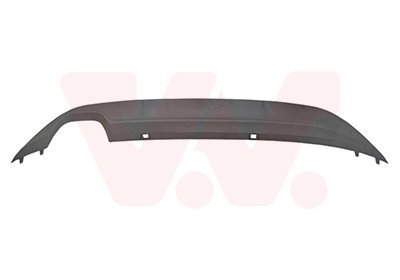### Search a car part :

Search by model
Search car part by reference# Spoiler VAN WEZEL 5766508

#### Ref: 5766508Shipped within 24 to 48 hours

#### 37.88 €TTC

Fitting Position : Rear
Exhaust System : Double End Pipe
Quality : Equipart

• VW GOLF VII (5G1, BQ1, BE1, BE2) 1.0 TSI
• VW GOLF VII (5G1, BQ1, BE1, BE2) 1.2 TSI
• VW GOLF VII (5G1, BQ1, BE1, BE2) 1.2 TSI
• VW GOLF VII (5G1, BQ1, BE1, BE2) 1.2 TSI
• VW GOLF VII (5G1, BQ1, BE1, BE2) 1.4 TGI CNG
• VW GOLF VII (5G1, BQ1, BE1, BE2) 1.4 TSI
• VW GOLF VII (5G1, BQ1, BE1, BE2) 1.4 TSI
• VW GOLF VII (5G1, BQ1, BE1, BE2) 1.4 TSI
• VW GOLF VII (5G1, BQ1, BE1, BE2) 1.4 TSI
• VW GOLF VII (5G1, BQ1, BE1, BE2) 1.4 TSI MultiFuel
• VW GOLF VII (5G1, BQ1, BE1, BE2) 1.4 TSI MultiFuel
• VW GOLF VII (5G1, BQ1, BE1, BE2) 1.6
• VW GOLF VII (5G1, BQ1, BE1, BE2) 1.6 TDI
• VW GOLF VII (5G1, BQ1, BE1, BE2) 1.6 TDI
• VW GOLF VII (5G1, BQ1, BE1, BE2) 1.6 TDI
• VW GOLF VII (5G1, BQ1, BE1, BE2) 1.6 TDI 4motion
• VW GOLF VII (5G1, BQ1, BE1, BE2) 1.6 TDI 4motion
• VW GOLF VII (5G1, BQ1, BE1, BE2) 2.0 GTI
• VW GOLF VII (5G1, BQ1, BE1, BE2) 2.0 TDI
• VW GOLF VII (5G1, BQ1, BE1, BE2) 2.0 TDI
• VW GOLF VII (5G1, BQ1, BE1, BE2) 2.0 TDI
• VW GOLF VII (5G1, BQ1, BE1, BE2) 2.0 TDI 4motion
• VW GOLF VII (5G1, BQ1, BE1, BE2) 1.0 TSI

DIEDERICHS : 2216068
PRASCO : VG4001861